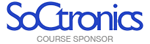Select Page

# SAMPLE QUESTIONS – Analog Design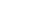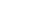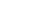1. ###### For the circuit shown below plot the trans-conductance as a functions of VDS. 1M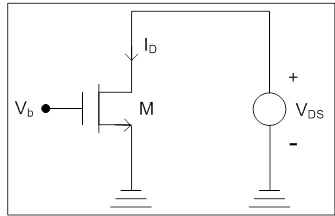5. ###### Sketch the drain current (ID) and transconductance (gm) of the transistor M as a function of input voltage. 2M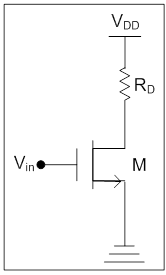6. Assuming the value of the resistor as R, What are the effective resistance from source to drain of the following two transistors? 2M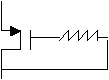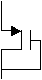7. Vin = Sin Ɯt. Find Vout  what happens if the input terminals are interchanged?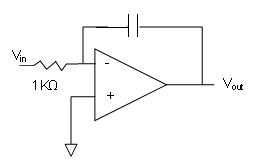3. ###### A 6KHz sinusoidal voltage is applied to a series RC circuit. The frequency of the voltage across the resistor is ________. 1M

4. Figure out the Vout wave form of the following circuits assuming R=1 K Ohm and C=1 micro-farad and input is square wave of Frequency 200Hz.  4M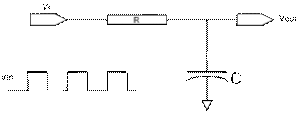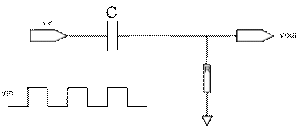5. ###### If x is 80% of y, then what percent of 2x is y ?